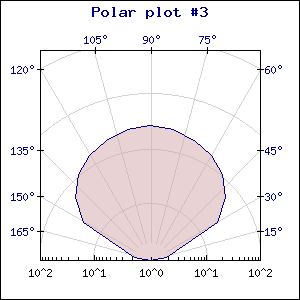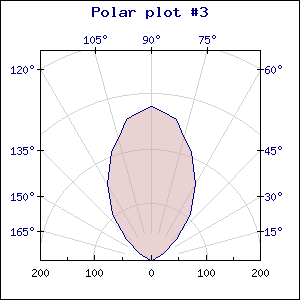Contents Previous Next

The radius axis can be shown in either a linear or logarithmic scale. This is controlled, as usual, by a call to PolarGraph::SetScale() The two examples below show the same plot in either linear or logarithmic scaleFigure 137: Using a logarithmic scale [src]Figure 138: Using a linear scale [src]

Please note that the maximum values of the scales are different.

By default the scale will be auto scaled depending on the data. You can also specify a manual scale by supplying an extra argument to the SetScale() value. The only difference from the manual scaling with the other X-Y-graphs is that for polar graph you only specify a manual maximum. The minimum will always be 0 for the linear scale and a scaled value of 10 (i.e 1, 0.1, 0.001 and so on) for the logarithmic scale.

The plot is clipped to the plot area so if you specify a smaller scale then the maximum value that part of the plot that are outside the plot area will be clipped.

Contents Previous Next